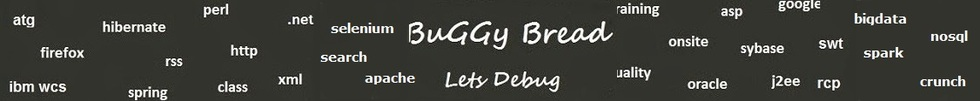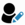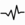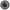Write a Program that gets a set of numbers , filters out the non prime numbers , calculate the factorial of each prime number and then finds the average of all factorials using Lambda expressions# Search Interview QuestionsMore than 3000 questions in repository.There are more than 900 unanswered questions.Have a video suggestion.
Click Correct / Improve and please let us know.
Label / Company      Label / Company / TextSubmit Question

Java - Interview Questions and AnswersQ1. What are the Wrapper classes available for primitive types ?Core JavaAnonymous
Ans. boolean - java.lang.Boolean
byte - java.lang.Byte
char - java.lang.Character
double - java.lang.Double
float - java.lang.Float
int - java.lang.Integer
long - java.lang.Long
short - java.lang.Short
void - java.lang.VoidHelp us improve. Please let us know the company, where you were asked this question :LikeDiscussCorrect / Improvejava   java5   data types   wrapper classes   adapter design patternrareRelated QuestionsWhat are wrapper classes ?What are the benefits of using Wrapper classes over primitive types ?What is the difference between uninitialized values for primitive types and Wrapper classes ?Difference between boolean and Boolean ?Explain Autoboxing ?What are Wrapper Classes ? What are Primitive Wrapper Classes ?What Design pattern Wrapper Classes implement ?Will this code give error if i try to add two heterogeneous elements in the arraylist. ? and Why ?Can we compare Integers by using equals() in Java ?Q2. Write a Program that gets a set of numbers , filters out the non prime numbers , calculate the factorial of each prime number and then finds the average of all factorials using Lambda expressions2017-05-14 14:02:57public static void main(String args[]) {

// Declare and Initialize the Collection
Set<Integer> intSet = new HashSet<Integer>();

double averageOfNonPrimeFactorials = intSet.stream().filter(p->checkIfPrime(p)).collect(Collectors.averagingInt(p->calculateFactorial(p)));

System.out.println(averageOfNonPrimeFactorials );
}

static private boolean checkIfPrime(int num){
for(int count=2;count < num;count++){
if(num % count == 0){
return false;
}
}
return true;
}

static private int calculateFactorial(int num){
int factorial = 1;
for(int count=num;count > 0;count--){
factorial = factorial * count;
}
return factorial;
}
}Help us improve. Please let us know the company, where you were asked this question :LikeDiscussCorrect / Improvejava 8  lambda expressions  lambda  filter  Collectors  factorial  prime numberRelated QuestionsWhat is a Lambda Expression ? What's its use ?Difference between final and effectively final ? Why is effectively final even required ?Difference between Predicate, Supplier and Consumer ?What are the problems one could face while working with serverless technologies like AWS Lambda ?How does AWS Lambda handle failure during event processing?What would you do if you see an error thrown by a lambda function as "Access Denied" while doing something with S3 ?What are the advantages and disadvantages of using Lambda over EC2 ?What are the different trigger types in AWS Lambda ?What does the following lambda expression means ?Difference between DoubleSummaryStatistics , IntSummaryStatistics and LongSummaryStatistics ?

## Help us and Others Improve. Please let us know the questions asked in any of your previous interview.

Any input from you will be highly appreciated and It will unlock the application for 10 more requests.